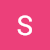# Estimating Expectations with Samples

The problem of computing an expectation of a function that takes a random variable as an input crops up in many areas of statistics and machine learning. There are numerous ways of estimating this expectation such as by using Markov Chain Monte Carlo (MCMC) methods or by using variational inference. In this short read, I’ll discuss the idea of using samples to estimate expectations and introduce one such MCMC method — Gibbs sampling — and how it can be used to estimate these expectations. But first, a bit on notation…

We say samples of a random variable x is drawn…